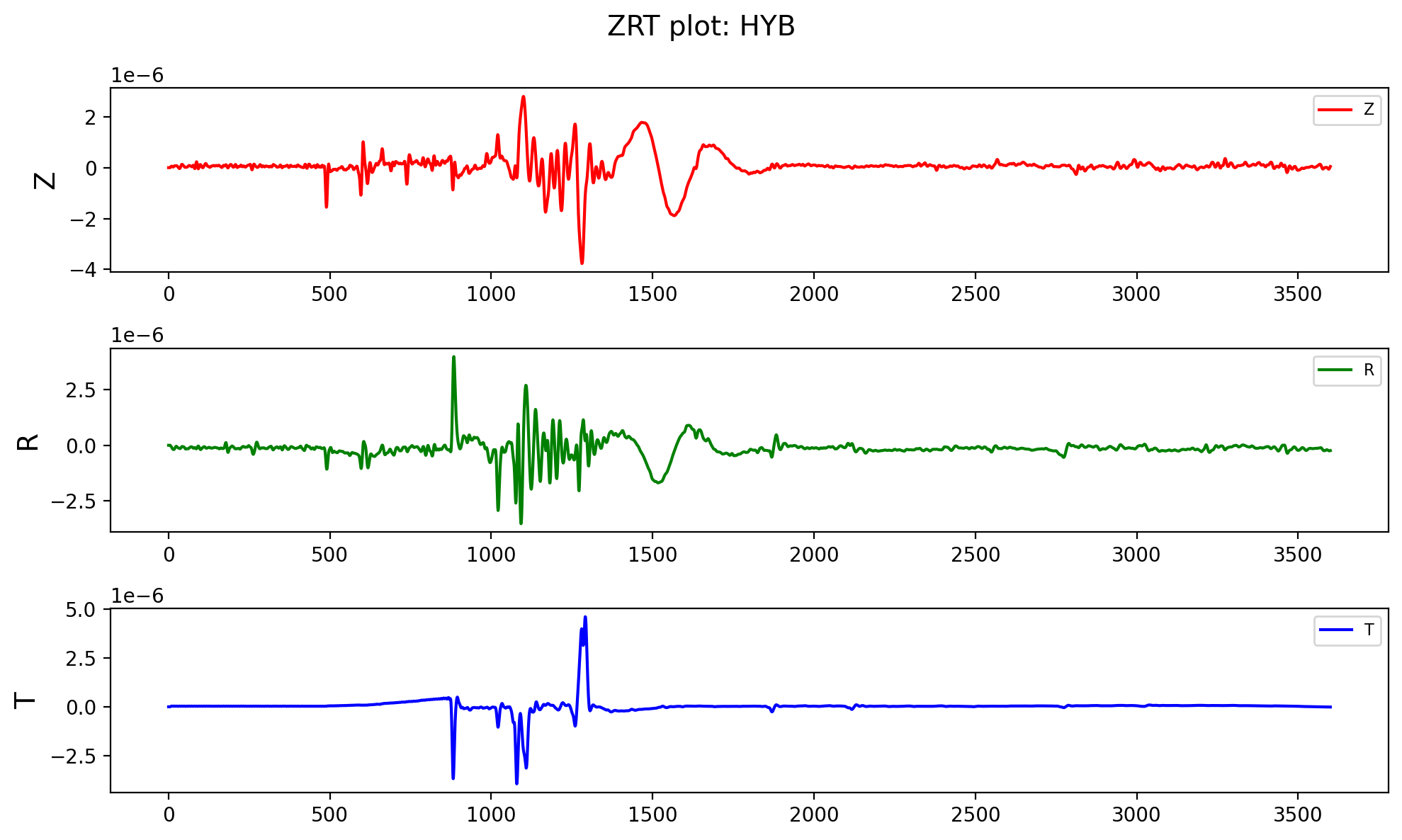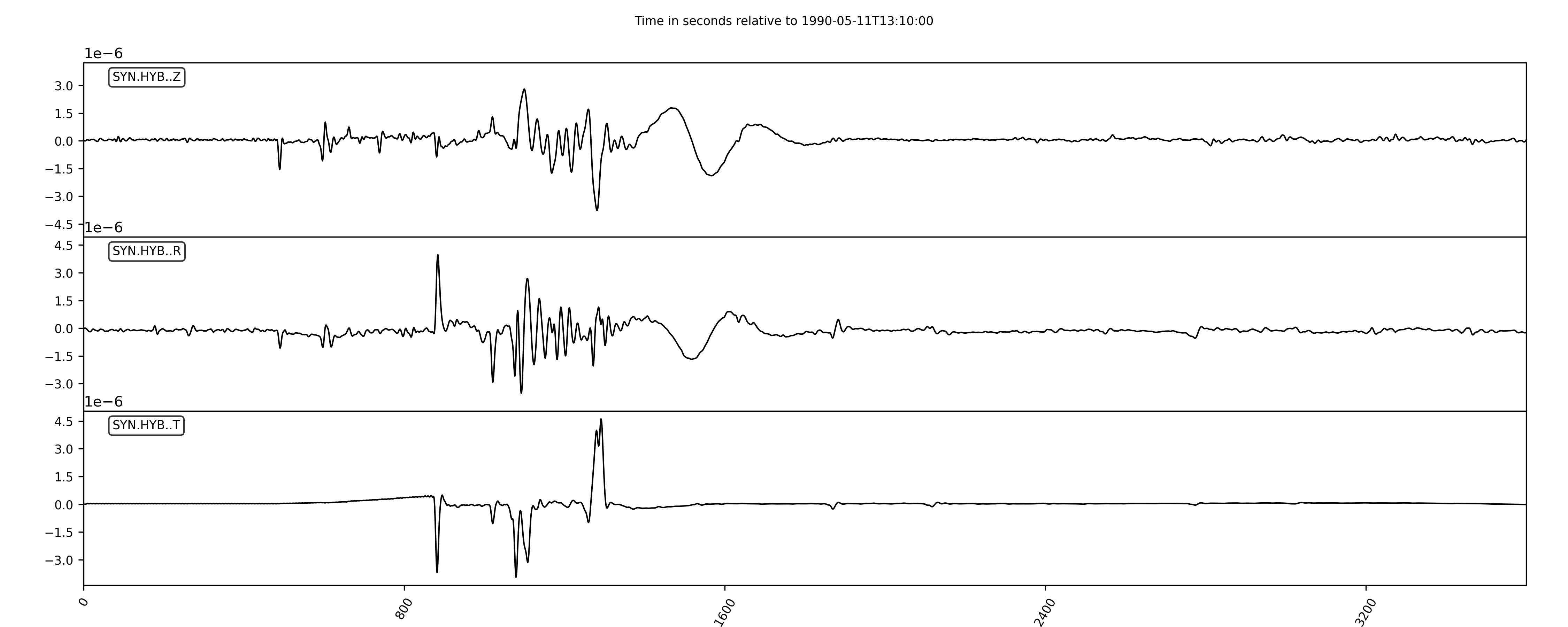# Write ASCII data to MSEED file using Obspy (codes included)

In this post, I will read a ASCII file whose first few lines contains the header information and then the three-component data. I will read using the pandas dataframe and then save it into mseed file using obspy. The header information will also be written into the file.

## Introduction

This post is about reading an ASCII file whose first few lines contain the header information and then the three-component data, and then finally writing it into the Mseed (or SAC) formatted file. I will read the data using the pandas dataframe and then save it into the mseed file using obspy. The header information will also be written into the file.

The ASCII file I used for this post has the following format:

199005111310
41.49000  130.42000 586.00 24.  12.630  -0.690 -11.940   3.930 -19.500 -12.270   6.80   5.00
HYB
17.41700   78.55300  510.00000  50.2354 257.5201
3 ZRT
3601     0.000
0.000  0.00000000E+00  0.00000000E+00  0.00000000E+00
1.000  0.00000000E+00  0.00000000E+00  0.00000000E+00
2.000  0.00000000E+00  0.00000000E+00  0.00000000E+00
3.000  0.00000000E+00  0.00000000E+00  0.00000000E+00
4.000  0.00000000E+00  0.00000000E+00  0.00000000E+00
5.000  0.00000000E+00  0.00000000E+00  0.00000000E+00
6.000  0.20651668E-07 -0.17604642E-07  0.12335463E-07
7.000  0.33964160E-07 -0.32352548E-07  0.20258623E-07
8.000  0.47860410E-07 -0.52818472E-07  0.28573858E-07
9.000  0.57876023E-07 -0.77652109E-07  0.34789229E-07
10.000  0.60017179E-07 -0.10427422E-06  0.36822529E-07
11.000  0.53305714E-07 -0.12976660E-06  0.34412122E-07
12.000  0.40943924E-07 -0.15182159E-06  0.29569563E-07
13.000  0.29080783E-07 -0.16921048E-06  0.25574463E-07
14.000  0.23762603E-07 -0.18157713E-06  0.25058633E-07
15.000  0.27860320E-07 -0.18878092E-06  0.28412138E-07
16.000  0.39770686E-07 -0.19026609E-06  0.33568093E-07
17.000  0.54573346E-07 -0.18492088E-06  0.37330729E-07
18.000  0.66849783E-07 -0.17162138E-06  0.37408331E-07
19.000  0.73454726E-07 -0.15025366E-06  0.33857066E-07
20.000  0.74688101E-07 -0.12267228E-06  0.29021215E-07
21.000  0.73373968E-07 -0.92971096E-07  0.26016018E-07
22.000  0.72622052E-07 -0.66704201E-07  0.26728559E-07
23.000  0.73745417E-07 -0.49206103E-07  0.30615360E-07
...


## Reading header from the first few lines of the ASCII file

As we can see, the first six lines of the ASCII file contains the header information. The first line is the event id following the origin-time format; the second line is the moment tensor solutions; the third line has the station and event info.

## Similar posts

#### Computing cross-correlation and spectrogram of two seismic traces (codes included)

We use the python open method to read that info into the memory to finally write into the mseed file.

import matplotlib.pyplot as plt
import numpy as np
import pandas as pd
import os

dataFileLoc = "OutputWaveforms" #location of the ascii file
dataFileName = "199005111310.HYB.ASC" #ascii file name

dataFile = os.path.join(dataFileLoc, dataFileName)

with open(dataFile, 'r') as ff:
eventid=line.strip()
cnt = 1
while line:
print("Line {}: {}".format(cnt, line.strip()))
cnt += 1

if cnt==2:
mtsol = line.strip()
elif cnt==3:
stnName = line.strip()
elif cnt==4:
stnLocs = line.strip()
elif cnt==6:
dataCounts = line.strip()
elif cnt>6:
break


## Read the three-component data using the Pandas DataFrame

In the above description of the ASCII data file, we can see that the data is stored in four columns, with the first shows the timestamps, and then the next three columns are the “Z”, “R”, “T”. We can easily read it using the pandas dataframe.

data_df = pd.read_csv(dataFile, skiprows=6, names= ['timestamp', 'Z', 'R', 'T'], sep='\s+', dtype={'Z': np.float64, "R": np.float64, "T": np.float64})


Notice that we have skipped the first six lines as those contain the header info, not the data.

## Quick plot of the data using matplotlib

Since we have loaded our data into the pandas dataframe, plotting the data is super simple.

fig, (ax1, ax2, ax3) = plt.subplots(3,1, figsize=(10,6))

ax1.plot(data_df['timestamp'], data_df['Z'], color="r", label="Z")
ax1.set_ylabel("Z", fontsize=14)
ax1.legend(fontsize=8, frameon=True)

plt.suptitle(f"ZRT plot: {stnName}", fontsize=14)

ax2.plot(data_df['timestamp'], data_df['R'], color="g", label="R")
ax2.set_ylabel("R", fontsize=14)
ax2.legend(fontsize=8, frameon=True)

ax3.plot(data_df['timestamp'], data_df['T'], color="b", label="T")
ax3.set_ylabel("T", fontsize=14)
ax3.legend(fontsize=8, frameon=True)

plt.tight_layout()

plt.savefig(os.path.join(dataFileLoc, f"{stnName}-ZRT.png"),
bbox_inches="tight",
dpi=200,
)
plt.close('all')## Writing the data to mseed file

To write the data to the mseed file through obspy, we first need to load the data into the obspy object Stream, and then saving and plotting the data is straightforward.

from obspy import Stream, Trace
from obspy.core import UTCDateTime

statsZ = {}
statsZ['network'] = "SYN" #fake network name
statsZ['station'] = stnName
# statsZ['npts'] = dataCounts.split()
statsZ['component'] = 'Z'

## Station location
statsZ['stla'] = stnLocs.split()
statsZ['stlo'] = stnLocs.split()

## Event location
statsZ['evla'] = stnLocs.split()
statsZ['evlo'] = stnLocs.split()
statsZ['evdp'] = stnLocs.split()

statsZ['starttime'] = UTCDateTime(eventid)
trZ = Trace(data=data_df['Z'].values, header = statsZ)

statsR = statsZ
statsR['component'] = 'R'
trR = Trace(data=data_df['R'].values, header = statsR)

statsT = statsZ
statsT['component'] = 'T'
trT = Trace(data=data_df['T'].values, header = statsT)

st = Stream(traces=[trZ, trR, trT])

print(st)

## Write the stream to file
st.write(os.path.join(dataFileLoc, f"{stnName}-ZRT-obspy.mseed"), format="MSEED")


We first used the header info from the ASCII file and wrote that into dictionaries (one for each component), and then we can write the data and the header information using the Trace object of the obspy. Then, we combine the three Trace objects into one stream. Next, we wrote the stream to the mseed file. We can quickly write to any other formats like SAC or others by just changing filename with the different extensions. obspy is smart enough to understand the different formats.

## Plot the Obspy stream and save it to the file

Finally, we can also plot the obspy stream using the plot method and then save it into the file.

## Plot the stream
stFig = st.plot(show=False,
size=(1500,600), number_of_ticks=6,
type='relative', tick_rotation=60, handle=True,
linewidth = 1)
plt.savefig(os.path.join(dataFileLoc, f"{stnName}-ZRT-obspy.png"), dpi=300)## Complete Script

Tags:

Categories:

Created on:#### Disclaimer of liability

The information provided by the Earth Inversion is made available for educational purposes only.

Whilst we endeavor to keep the information up-to-date and correct. Earth Inversion makes no representations or warranties of any kind, express or implied about the completeness, accuracy, reliability, suitability or availability with respect to the website or the information, products, services or related graphics content on the website for any purpose.

UNDER NO CIRCUMSTANCE SHALL WE HAVE ANY LIABILITY TO YOU FOR ANY LOSS OR DAMAGE OF ANY KIND INCURRED AS A RESULT OF THE USE OF THE SITE OR RELIANCE ON ANY INFORMATION PROVIDED ON THE SITE. ANY RELIANCE YOU PLACED ON SUCH MATERIAL IS THEREFORE STRICTLY AT YOUR OWN RISK.